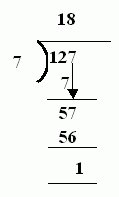Name: ___________________Date:___________________

 Email us to get an instant 20% discount on highly effective K-12 Math & English kwizNET Programs!

### MEAP Preparation - Grade 4 Mathematics1.3 Division With Remainders

 Look at the larger number which is the dividend. The lesser number is called divisor. The dividend is divided by the divisor. If the divisor is not exactly divisible by the divisor, it results in a remainder. Example: 127/718 times 7 gives 126. Answer: 18R1 Directions: Divide the following. Also write at least 10 examples of your own.
 Q 1: Which is the correct answer to 84/9?9R38R2none of these7R6 Q 2: Which is the correct answer to 48/9 ?none of these5 R36 R 33R5 Q 3: Which is the correct answer to 22/4?none of these5R25R13R2 Q 4: Which is the correct answer to 27/5?5R24R3none of these5R1 Q 5: Which is the correct answer to 66/8?7R58R28R1none of these Q 6: Which is the correct answer to 14/3?none of these4R23R22R1 Q 7: Which is the correct answer to 25/38R1none of these7R47R3 Q 8: Which is the correct answer to 75/8?9R18R49R3none of these Question 9: This question is available to subscribers only! Question 10: This question is available to subscribers only!